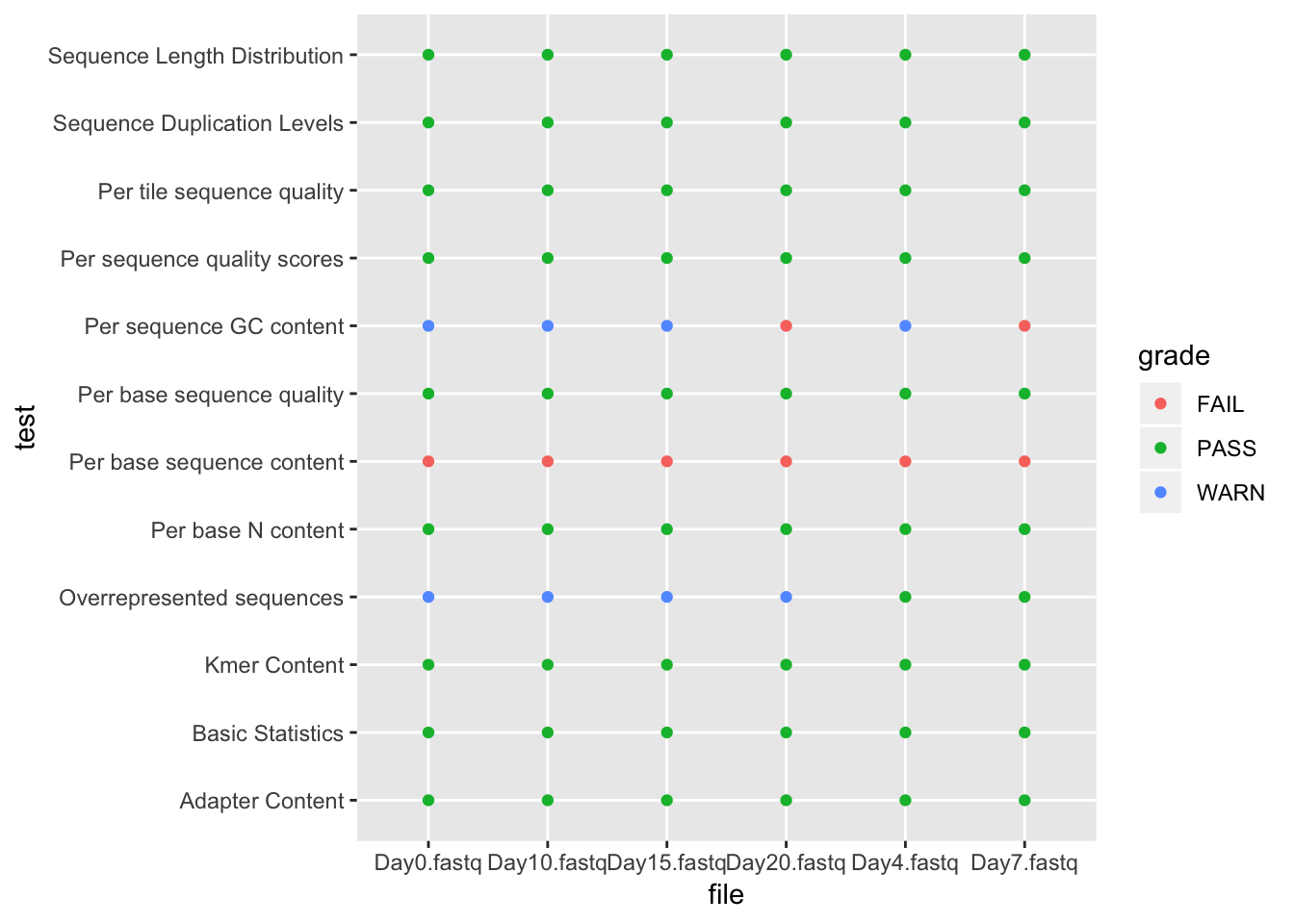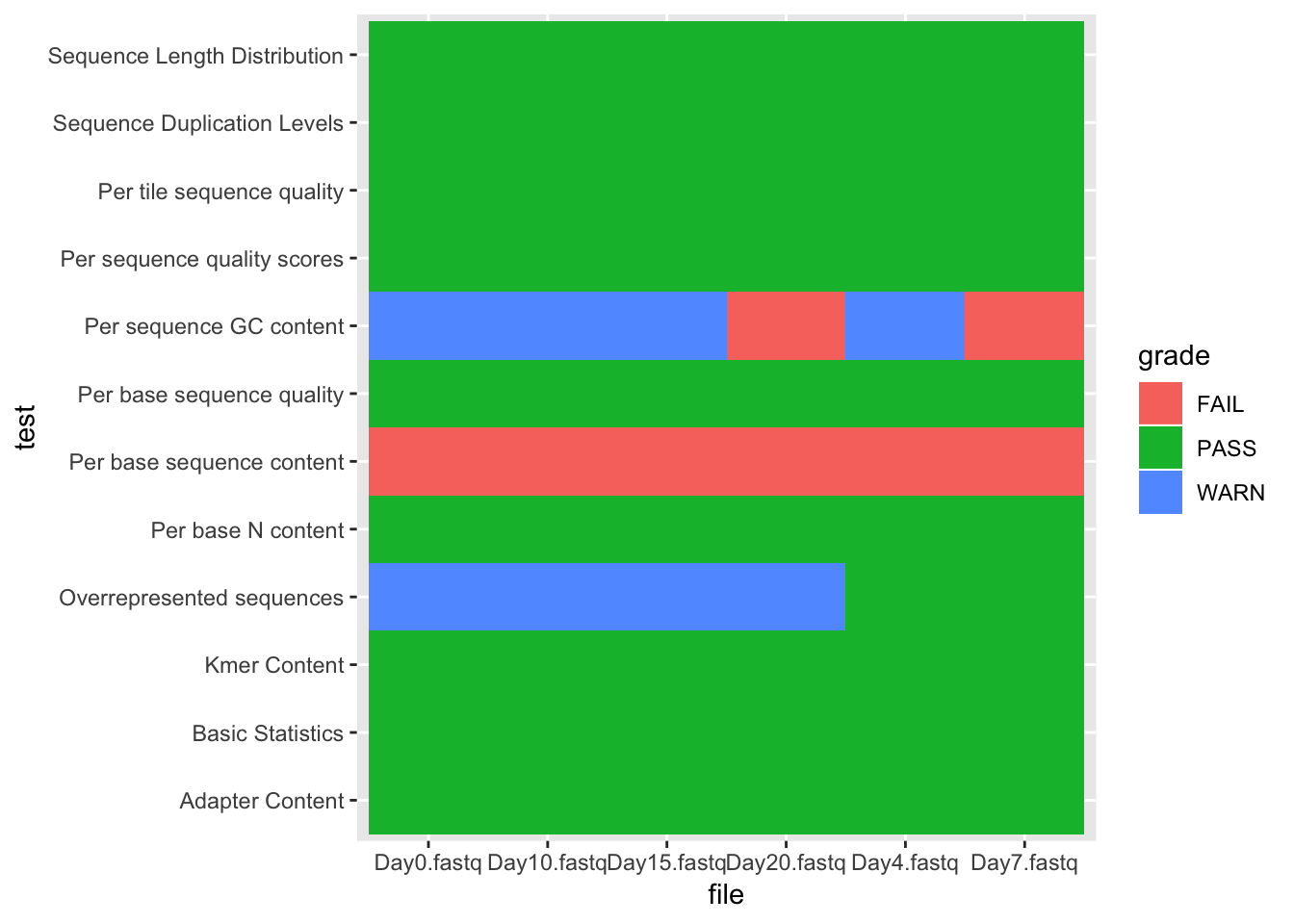This lesson covers packages primarily by Hadley Wickham for tidying data and then working with it in tidy form, collectively known as the “tidyverse”.

The packages we are using in this lesson are all from CRAN, so we can install them with `install.packages`. Don’t run this if you are using our biotraining server, the packages are already installed!

``````# install.packages(c(
#     "tidyverse",
#     "viridis",
#     "broom"
# ))``````
``````library(tidyverse) # Load all "tidyverse" libraries.
# OR
# library(tidyr)   # Data frame tidying functions.
# library(dplyr)   # General data frame manipulation.
# library(ggplot2) # Flexible plotting.

library(viridis)   # Viridis color scale.``````

These packages usually have useful documentation in the form of “vignettes”. These are readable on the CRAN website, or within R:

``````vignette()
vignette(package="dplyr")
vignette("dplyr", package="dplyr")``````

Let’s continue our examination of the FastQC output. If you’re starting fresh for this lesson, you can load the necessary data frame with:

``bigtab <- read_csv("r-more-files/fastqc.csv")``
``````## Parsed with column specification:
## cols(
##   test = col_character(),
##   file = col_character()
## )``````

# ggplot2 revisited

We saw ggplot2 in the introductory R day. Recall that we could assign columns of a data frame to aesthetics–x and y position, color, etc–and then add “geom”s to draw the data.

With `ggplot2` we can easily view the whole data set.

``````ggplot(bigtab, aes(x=file,y=test,color=grade)) +
geom_point()``````With categorical data on the x and y axes, a better geom to use is `geom_tile`.

``````ggplot(bigtab, aes(x=file,y=test,fill=grade)) +
geom_tile()``````## Publication quality images

`ggplot2` offers a very wide variety of ways to adjust a plot. For categorical aesthetics, usually the first step is ensuring the relevant column is a factor with a meaningful level order.

``````y_order <- sort(unique(bigtab\$test), decreasing=T)  # y axis plots from bottom to top, so reverse
bigtab\$test <- factor(bigtab\$test, levels=y_order)

x_order <- unique(bigtab\$file)
bigtab\$file <- factor(bigtab\$file, levels=x_order)

# Only necessary if not continuing from previous lesson on programming!
color_order <- c("FAIL", "WARN", "PASS")